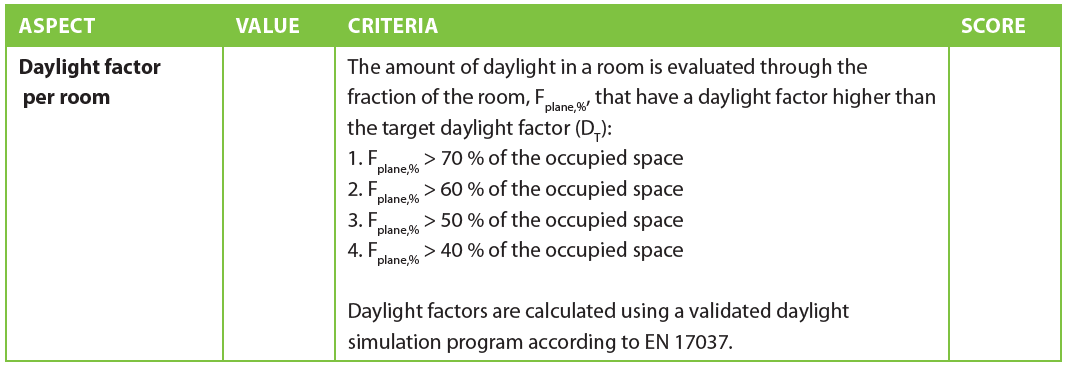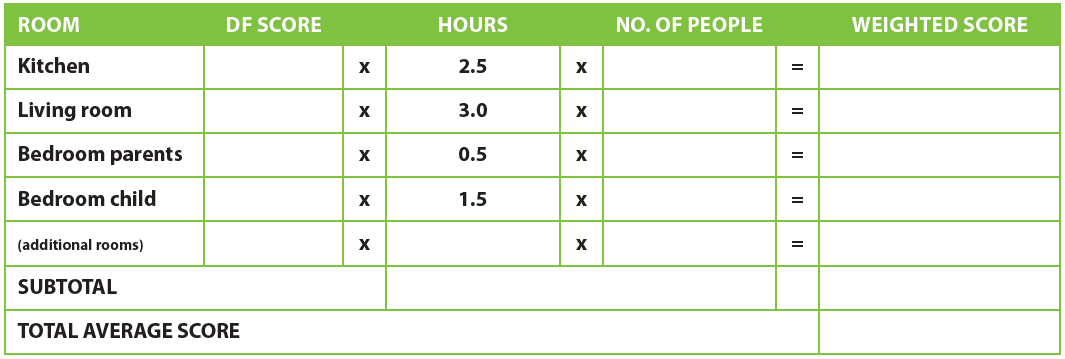Building geometry, surrounding landscape, neighboring buildings and surface properties (color, diffuseness, specularity, transmittance, reflectance) are basic boundary conditions to assess daylight factor (DF), as the main quantitative classification of a project.

The calculation method of option 1 is based on the daylight factor method (method 1) in EN 17037.

• The amount of daylight in a room is evaluated using daylight factors on a horizontal work plane divided into a grid with a height above floor level of 0.85 m.

• Daylight factors must be calculated with a daylight simulation program validated against CIE 16:1970. The used sky type should be TYPE 1 or TYPE 16 from the ISO 15469:2004 and it should be stated what type is used in the calculations.

• The evaluation includes the living and activity zones (such as living room, workspace, dining room, kitchen, bedroom or children’s room).

• Half of the daylit hours should have a fraction of space for the target level above the specified value for each category. Meaning that the daylight factor for each grid point should be higher than the daylight factor target level for a specific fraction of the grid, e.g. 60%.D_T depends on location and by that the median external diffuse illuminance E_{v,d,med}. Values of E_{v,d,med} for different nations / capitals are shown in Annex 1 together with the corresponding values of D_T.E_{v,d,med} can be calculated using hourly values of the diffuse horizontal illuminance from the sky using yearly weather data. E_{v,d,med} is median of the 4380 highest hourly values, equivalent to the 75th percentile of the yearly data.

Total average score = total weighted score/total hours use

The total average score is based on multiplying the score for each room with the amount of hours spent and the number of people in that room during daylight hours, then dividing by the total number of people-hours (see also table 1 on page 10).
In case no actual or predicted use numbers are available, use the default use hours in the table below. This way, the rooms that are being used while there is daylight, such as the living room, will contribute with higher weight than, for example, the master bedroom, which is used by adults mostly during the night.